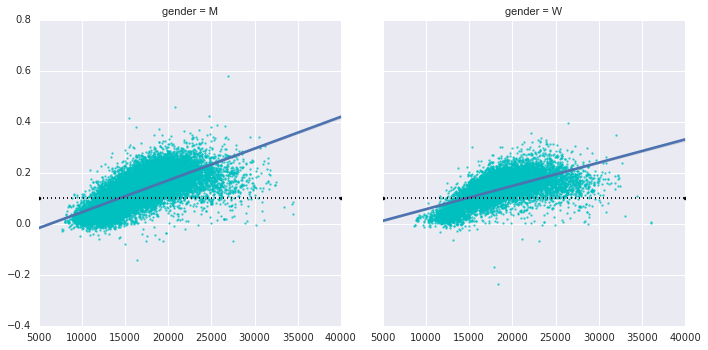# 8.17 使用 Seaborn 的可视化

Matplotlib 据证明是一种非常有用和流行的可视化工具，但即使狂热的用户也会承认它经常会有很多不足之处。有几个对 Matplotlib 的有效的抱怨常常出现：

• 在 2.0 版之前，Matplotlib 的默认值并不是最佳选择。 它基于大约 1999 年的 MATLAB，经常是这样。
• Matplotlib 的 API 相对较低。 可以进行复杂的统计可视化，但通常需要大量的样板代码。
• Matplotlib 比 Pandas 早了十多年，因此不适合与 Pandas 的`DataFrame`一起使用。 为了可视化来自 Pandas`DataFrame`的数据，你必须提取每个`Series``并经常将它们连接成正确的格式。 如果有一个绘图库可以智能地在绘图中使用`DataFrame`标签会更好。

## Seaborn VS Matplotlib

``````import matplotlib.pyplot as plt
plt.style.use('classic')
%matplotlib inline
import numpy as np
import pandas as pd
``````

``````# 创建一些数据
rng = np.random.RandomState(0)
x = np.linspace(0, 10, 500)
y = np.cumsum(rng.randn(500, 6), 0)
``````

``````# 使用 Matplotlib 默认值绘制数据
plt.plot(x, y)
plt.legend('ABCDEF', ncol=2, loc='upper left');
``````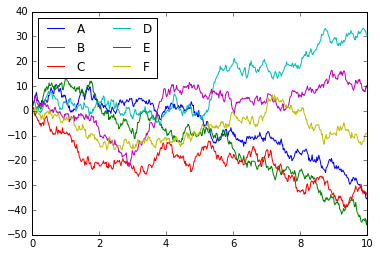``````import seaborn as sns
sns.set()
``````

``````# 和上面一样的绘图代码
plt.plot(x, y)
plt.legend('ABCDEF', ncol=2, loc='upper left');
``````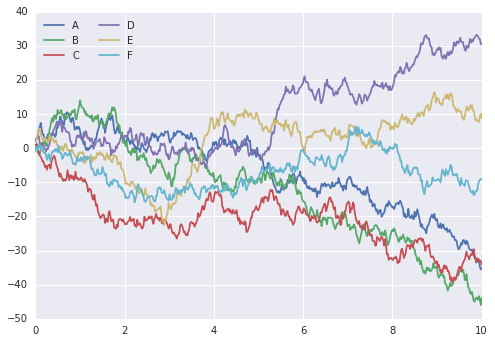## 探索 Seaborn 绘图

Seaborn 的主要思想是它提供高级命令，来创建用于统计数据探索，甚至是一些统计模型拟合的各种绘图类型。

### 直方图，KDE，和密度

``````data = np.random.multivariate_normal([0, 0], [[5, 2], [2, 2]], size=2000)
data = pd.DataFrame(data, columns=['x', 'y'])

for col in 'xy':
plt.hist(data[col], normed=True, alpha=0.5)
``````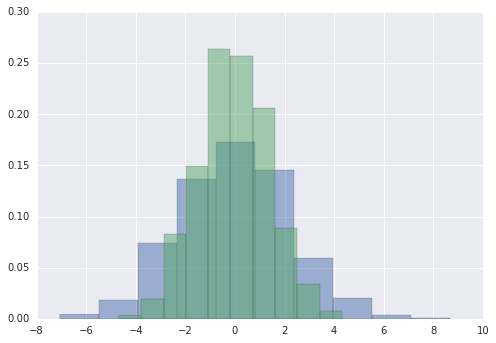``````for col in 'xy':
``````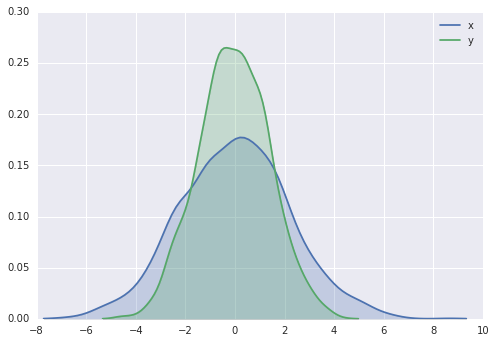``````sns.distplot(data['x'])
sns.distplot(data['y']);
``````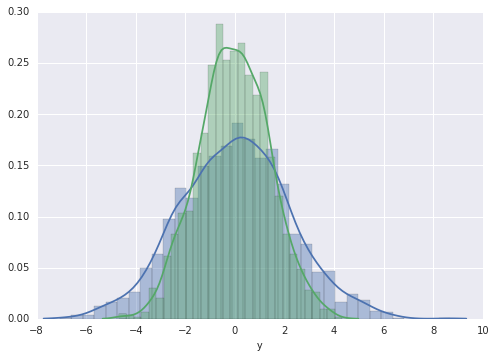``````sns.kdeplot(data);
``````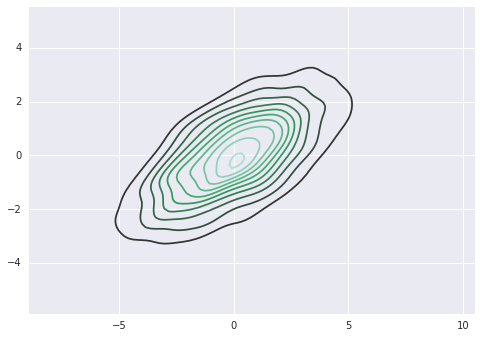``````with sns.axes_style('white'):
sns.jointplot("x", "y", data, kind='kde');
``````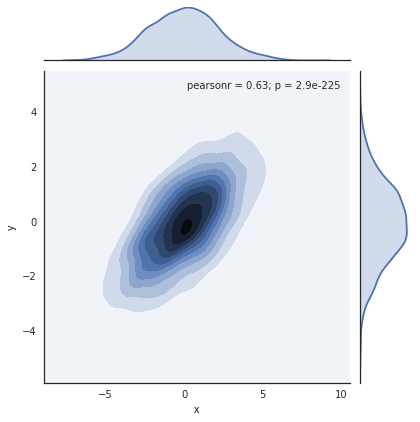``````with sns.axes_style('white'):
sns.jointplot("x", "y", data, kind='hex')
``````### 配对绘图

``````iris = sns.load_dataset("iris")
``````
sepal_length sepal_width petal_length petal_width species
0 5.1 3.5 1.4 0.2 setosa
1 4.9 3.0 1.4 0.2 setosa
2 4.7 3.2 1.3 0.2 setosa
3 4.6 3.1 1.5 0.2 setosa
4 5.0 3.6 1.4 0.2 setosa

``````sns.pairplot(iris, hue='species', size=2.5);
``````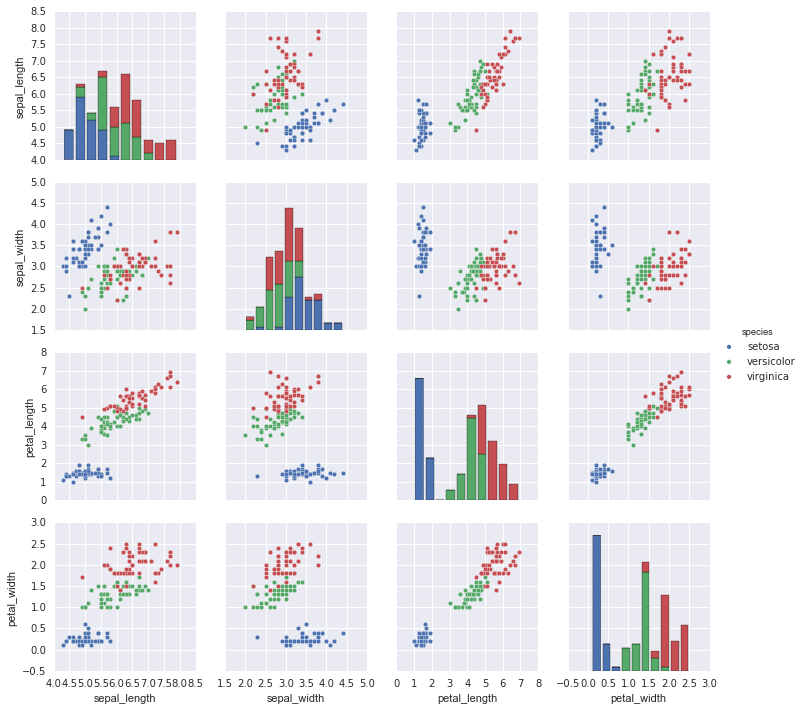### 分面直方图

``````tips = sns.load_dataset('tips')
``````
total_bill tip sex smoker day time size
0 16.99 1.01 Female No Sun Dinner 2
1 10.34 1.66 Male No Sun Dinner 3
2 21.01 3.50 Male No Sun Dinner 3
3 23.68 3.31 Male No Sun Dinner 2
4 24.59 3.61 Female No Sun Dinner 4
``````tips['tip_pct'] = 100 * tips['tip'] / tips['total_bill']

grid = sns.FacetGrid(tips, row="sex", col="time", margin_titles=True)
grid.map(plt.hist, "tip_pct", bins=np.linspace(0, 40, 15));
``````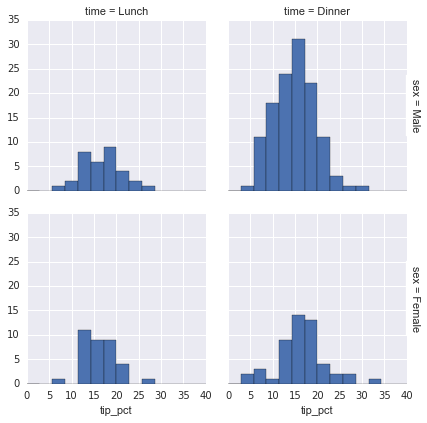### 因子图

``````with sns.axes_style(style='ticks'):
g = sns.factorplot("day", "total_bill", "sex", data=tips, kind="box")
g.set_axis_labels("Day", "Total Bill");
``````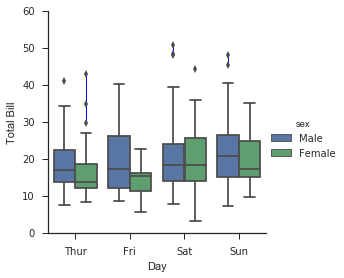### 联合分布

``````with sns.axes_style('white'):
sns.jointplot("total_bill", "tip", data=tips, kind='hex')
``````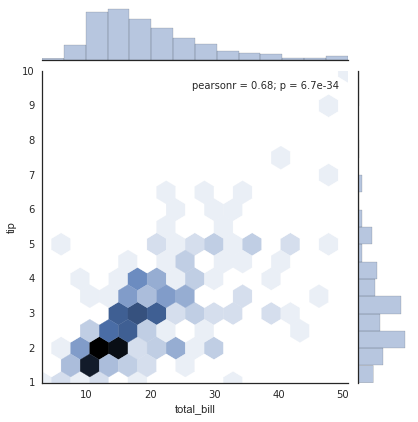``````sns.jointplot("total_bill", "tip", data=tips, kind='reg');
``````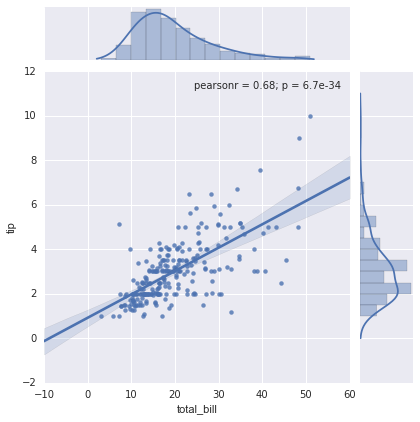### 条形图

``````planets = sns.load_dataset('planets')
``````
method number orbital_period mass distance year
0 Radial Velocity 1 269.300 7.10 77.40 2006
1 Radial Velocity 1 874.774 2.21 56.95 2008
2 Radial Velocity 1 763.000 2.60 19.84 2011
3 Radial Velocity 1 326.030 19.40 110.62 2007
4 Radial Velocity 1 516.220 10.50 119.47 2009
``````with sns.axes_style('white'):
g = sns.factorplot("year", data=planets, aspect=2,
kind="count", color='steelblue')
g.set_xticklabels(step=5)
``````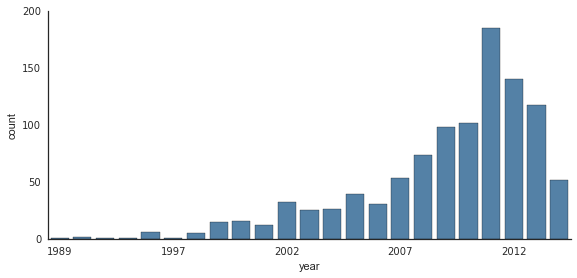``````with sns.axes_style('white'):
g = sns.factorplot("year", data=planets, aspect=4.0, kind='count',
hue='method', order=range(2001, 2015))
g.set_ylabels('Number of Planets Discovered')
``````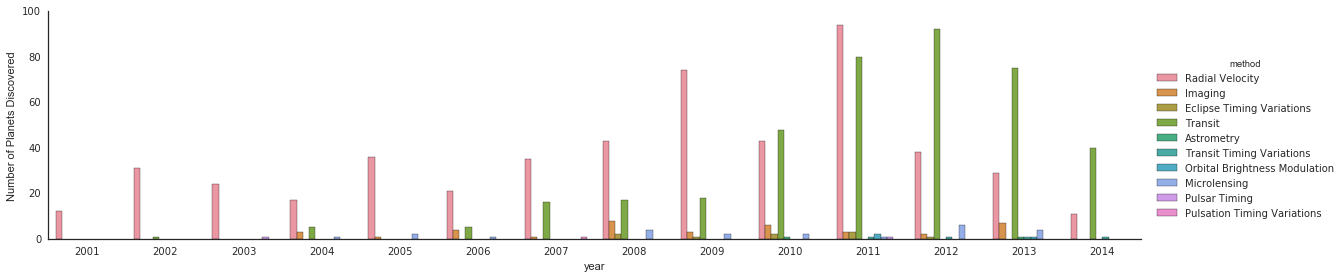## 示例：探索马拉松结束时间

``````# !curl -O https://raw.githubusercontent.com/jakevdp/marathon-data/master/marathon-data.csv

``````
age gender split final
0 33 M 01:05:38 02:08:51
1 32 M 01:06:26 02:09:28
2 31 M 01:06:49 02:10:42
3 38 M 01:06:16 02:13:45
4 31 M 01:06:32 02:13:59

``````data.dtypes

'''
age        int64
gender    object
split     object
final     object
dtype: object
'''
``````

``````def convert_time(s):
h, m, s = map(int, s.split(':'))
return pd.datetools.timedelta(hours=h, minutes=m, seconds=s)

converters={'split':convert_time, 'final':convert_time})
``````
age gender split final
0 33 M 01:05:38 02:08:51
1 32 M 01:06:26 02:09:28
2 31 M 01:06:49 02:10:42
3 38 M 01:06:16 02:13:45
4 31 M 01:06:32 02:13:59
``````data.dtypes

'''
age                 int64
gender             object
split     timedelta64[ns]
final     timedelta64[ns]
dtype: object
'''
``````

``````data['split_sec'] = data['split'].astype(int) / 1E9
data['final_sec'] = data['final'].astype(int) / 1E9
``````
age gender split final split_sec final_sec
0 33 M 01:05:38 02:08:51 3938.0 7731.0
1 32 M 01:06:26 02:09:28 3986.0 7768.0
2 31 M 01:06:49 02:10:42 4009.0 7842.0
3 38 M 01:06:16 02:13:45 3976.0 8025.0
4 31 M 01:06:32 02:13:59 3992.0 8039.0

``````with sns.axes_style('white'):
g = sns.jointplot("split_sec", "final_sec", data, kind='hex')
g.ax_joint.plot(np.linspace(4000, 16000),
np.linspace(8000, 32000), ':k')
``````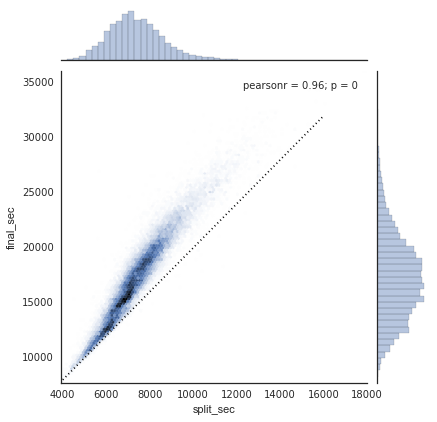``````data['split_frac'] = 1 - 2 * data['split_sec'] / data['final_sec']
``````
age gender split final split_sec final_sec split_frac
0 33 M 01:05:38 02:08:51 3938.0 7731.0 -0.018756
1 32 M 01:06:26 02:09:28 3986.0 7768.0 -0.026262
2 31 M 01:06:49 02:10:42 4009.0 7842.0 -0.022443
3 38 M 01:06:16 02:13:45 3976.0 8025.0 0.009097
4 31 M 01:06:32 02:13:59 3992.0 8039.0 0.006842

``````sns.distplot(data['split_frac'], kde=False);
plt.axvline(0, color="k", linestyle="--");
``````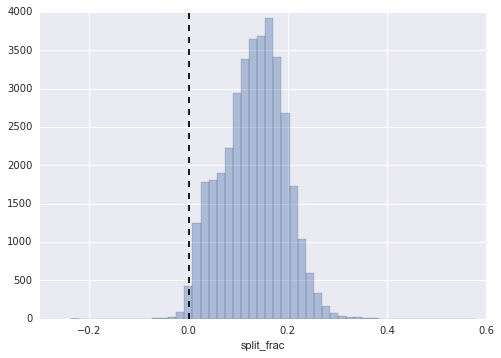``````sum(data.split_frac < 0)

# 251
``````

``````g = sns.PairGrid(data, vars=['age', 'split_sec', 'final_sec', 'split_frac'],
hue='gender', palette='RdBu_r')
g.map(plt.scatter, alpha=0.8)
````````````sns.kdeplot(data.split_frac[data.gender=='M'], label='men', shade=True)
plt.xlabel('split_frac');
````````````sns.violinplot("gender", "split_frac", data=data,
palette=["lightblue", "lightpink"]);
``````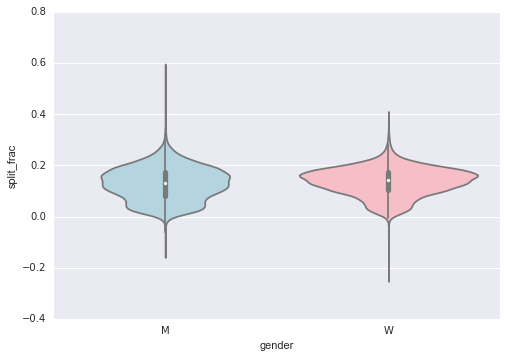``````data['age_dec'] = data.age.map(lambda age: 10 * (age // 10))
``````
age gender split final split_sec final_sec split_frac age_dec
0 33 M 01:05:38 02:08:51 3938.0 7731.0 -0.018756 30
1 32 M 01:06:26 02:09:28 3986.0 7768.0 -0.026262 30
2 31 M 01:06:49 02:10:42 4009.0 7842.0 -0.022443 30
3 38 M 01:06:16 02:13:45 3976.0 8025.0 0.009097 30
4 31 M 01:06:32 02:13:59 3992.0 8039.0 0.006842 30
``````men = (data.gender == 'M')
women = (data.gender == 'W')

with sns.axes_style(style=None):
sns.violinplot("age_dec", "split_frac", hue="gender", data=data,
split=True, inner="quartile",
palette=["lightblue", "lightpink"]);
``````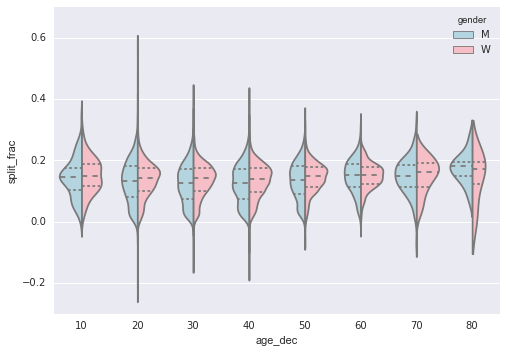``````(data.age > 80).sum()

# 7
``````

``````g = sns.lmplot('final_sec', 'split_frac', col='gender', data=data,
markers=".", scatter_kws=dict(color='c'))
g.map(plt.axhline, y=0.1, color="k", ls=":");
``````